Function Repository Resource:

# WolframModelGlocalBranchialGraph

Generate a graph of branch pair ancestry for a Wolfram model "glocal" (hybrid of global and local) multiway system

Contributed by: Jonathan Gorard
 ResourceFunction["WolframModelGlocalBranchialGraph"][rules,init,n] generates the graph of branch pair ancestry after n steps in the glocal multiway evolution of a Wolfram model system with the specified rules, starting from initial conditions init. ResourceFunction["WolframModelGlocalBranchialGraph"][rules→sel,init,n] uses the function sel to select which of the events obtained at each step to include in the evolution.

## Details and Options

Glocal multiway systems combine the global events (and the corresponding causal structure) of ordinary multiway systems, with the individual token philosophy of local multiway systems. A single event vertex in the evolution causal graph (or token event graph) for a glocal multiway system will, in general, have many incoming and outgoing evolution edges, corresponding to the fact that several tokens must be assembled together in order to reconstruct a single global input or output state for the event.
The branchial graph for a glocal multiway system shows the branch pair (i.e. critical pair) ancestry for a glocal evolution causal graph/token event graph. Unlike the branchial graph for an ordinary (global) multiway system, which only contains information regarding the evolution ancestry of states, glocal branchial graphs contain information about both the causal ancestry of events and the evolution ancestry of states/tokens.
Argument and option patterns for ResourceFunction["WolframModelGlocalBranchialGraph"] are similar to those of the resource function MultiwaySystem.
Replacement rules for Wolfram model systems are specified as {{{a1,a2,},{b1,b2,},}{{u1,u2,},},}.
ResourceFunction["WolframModelGlocalBranchialGraph"] accepts both individual rules and lists of rules, and likewise for initial conditions. ResourceFunction["WolframModelGlocalBranchialGraph"][rules,init,n] is interpreted as ResourceFunction["WolframModelGlocalBranchialGraph"][rules,{init},n], etc.
The event selection function sel in ResourceFunction["WolframModelGlocalBranchialGraph"][rulessel,] can have the following special forms:
 "Sequential" applies the first possible replacement (sequential substitution system) "Random" applies a random replacement {"Random",n} applies n randomly chosen replacements
Duplicated tokens are displayed separately at each time step by default, although this can be overridden by setting "DeduplicateTokens"True.
Events are represented in the form {rule,input,rest}, where rule is the instantiated rule used in the updating event, input is the part of the state to which the rule is applied and rest is the remainder of the state. For hypergraph substitution systems, rest is given in the form {prefix,suffix}.
Options for ResourceFunction["WolframModelGlocalBranchialGraph"] include:
 "DeduplicateTokens" False whether to merge all instances of equivalent tokens that appear at each time step "VertexRendering" True whether to use special rendering for state/token and event vertices "StateRenderingFunction" Automatic how to label states/tokens that appear in the evolution causal graph/token event graph "EventRenderingFunction" Automatic how to label events that appear in the evolution causal graph/token event graph
All of the standard options for Graph can also be applied to ResourceFunction["WolframModelGlocalBranchialGraph"].
Possible settings for "StateRenderingFunction" and "EventRenderingFunction" include:
 Automatic make a label from the name of the vertex Inherited use the explicit vertex name as the label None use no label for the vertex "string" use a shape from the VertexShapeFunction collection func apply the function func to the name of the vertex

## Examples

### Basic Examples (3)

Generate glocal branchial graphs for two simple Wolfram model glocal multiway systems:

 In:=Out=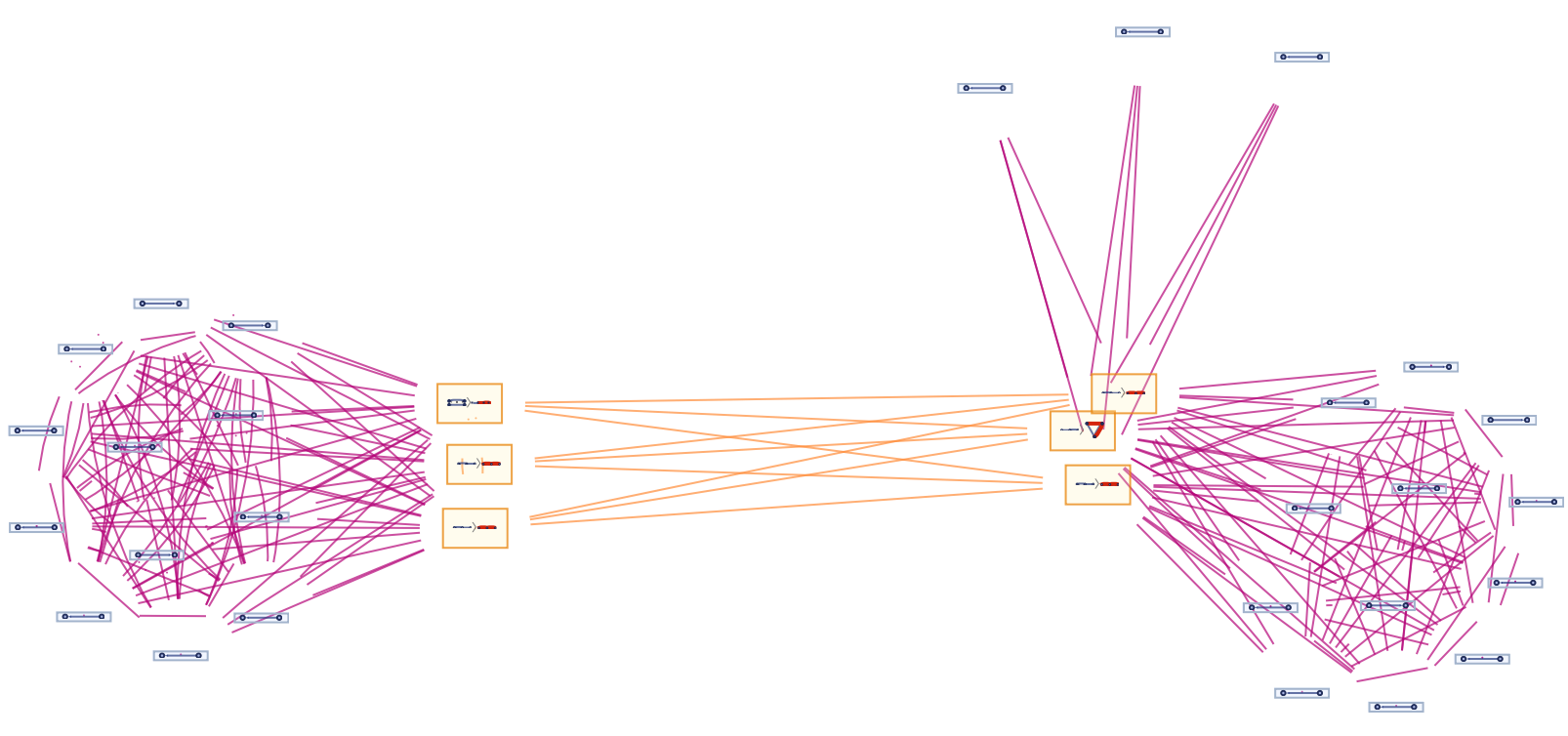In:=Out=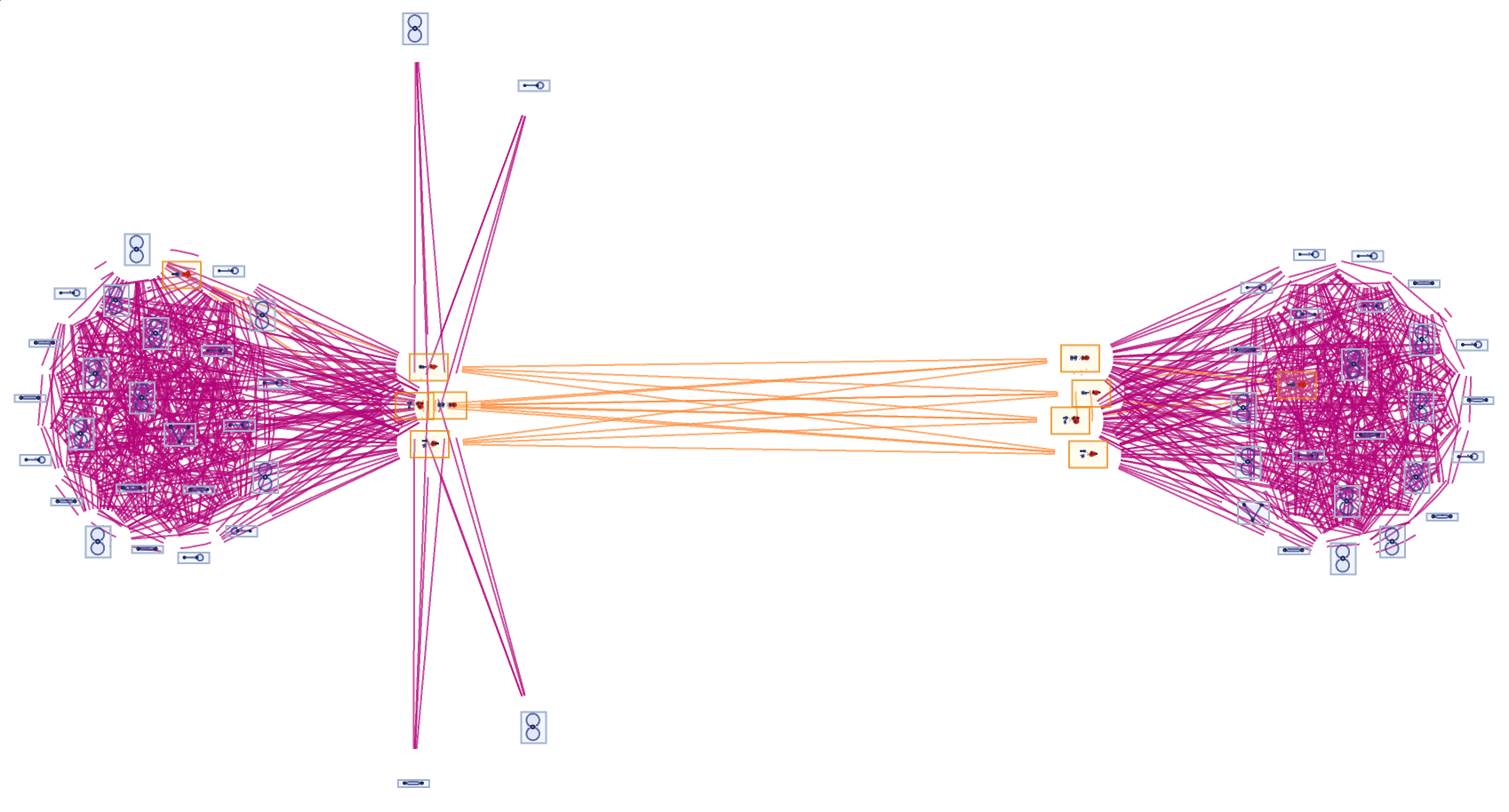Show just the structure of the graphs, without labels:

 In:=Out=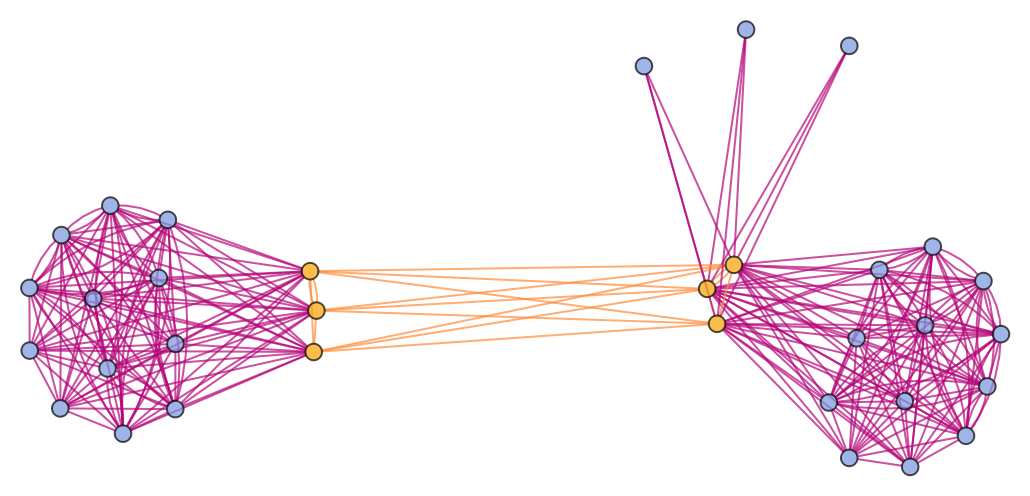In:=Out=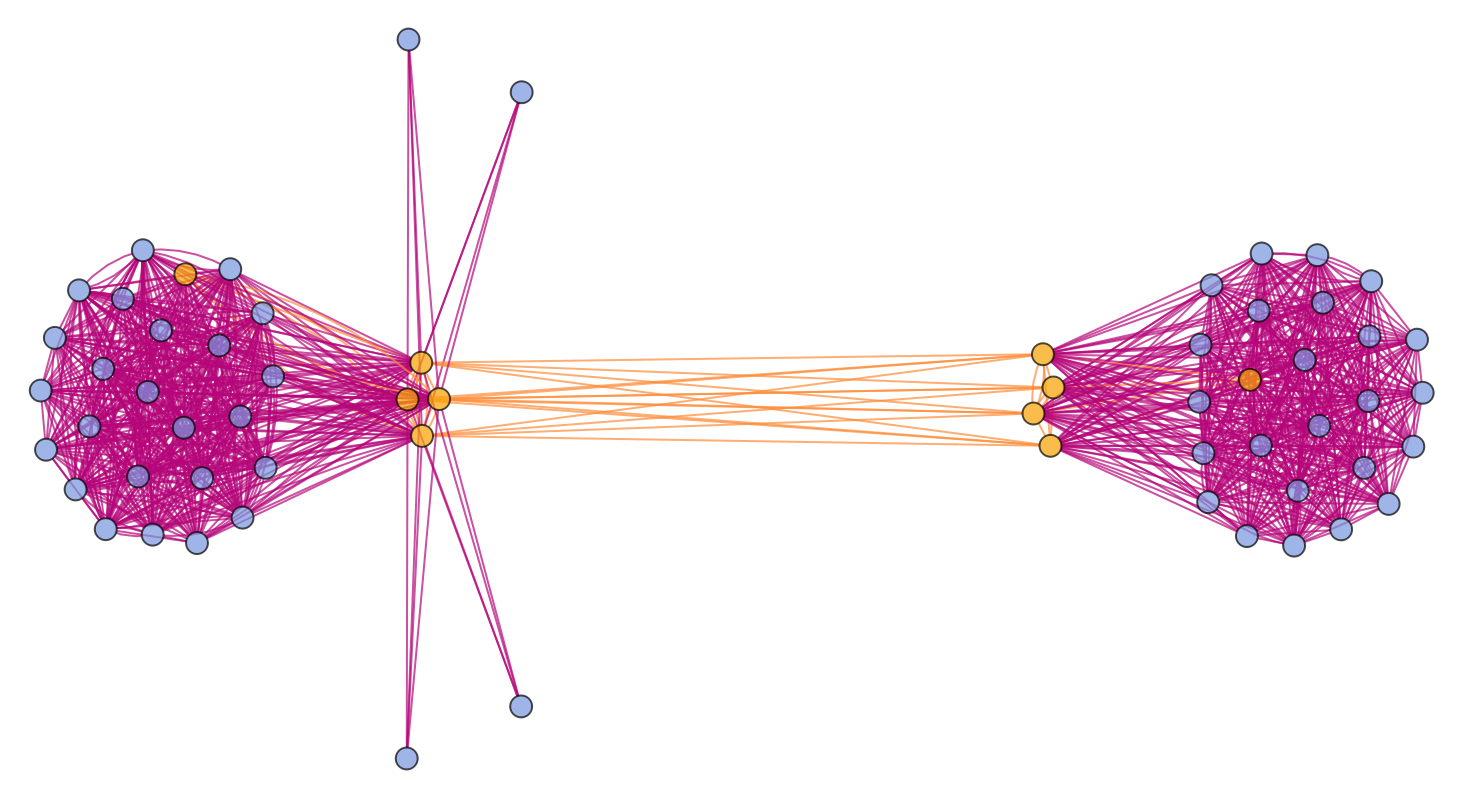Generate a glocal branchial graph for a more complicated Wolfram model evolution:

 In:=Out=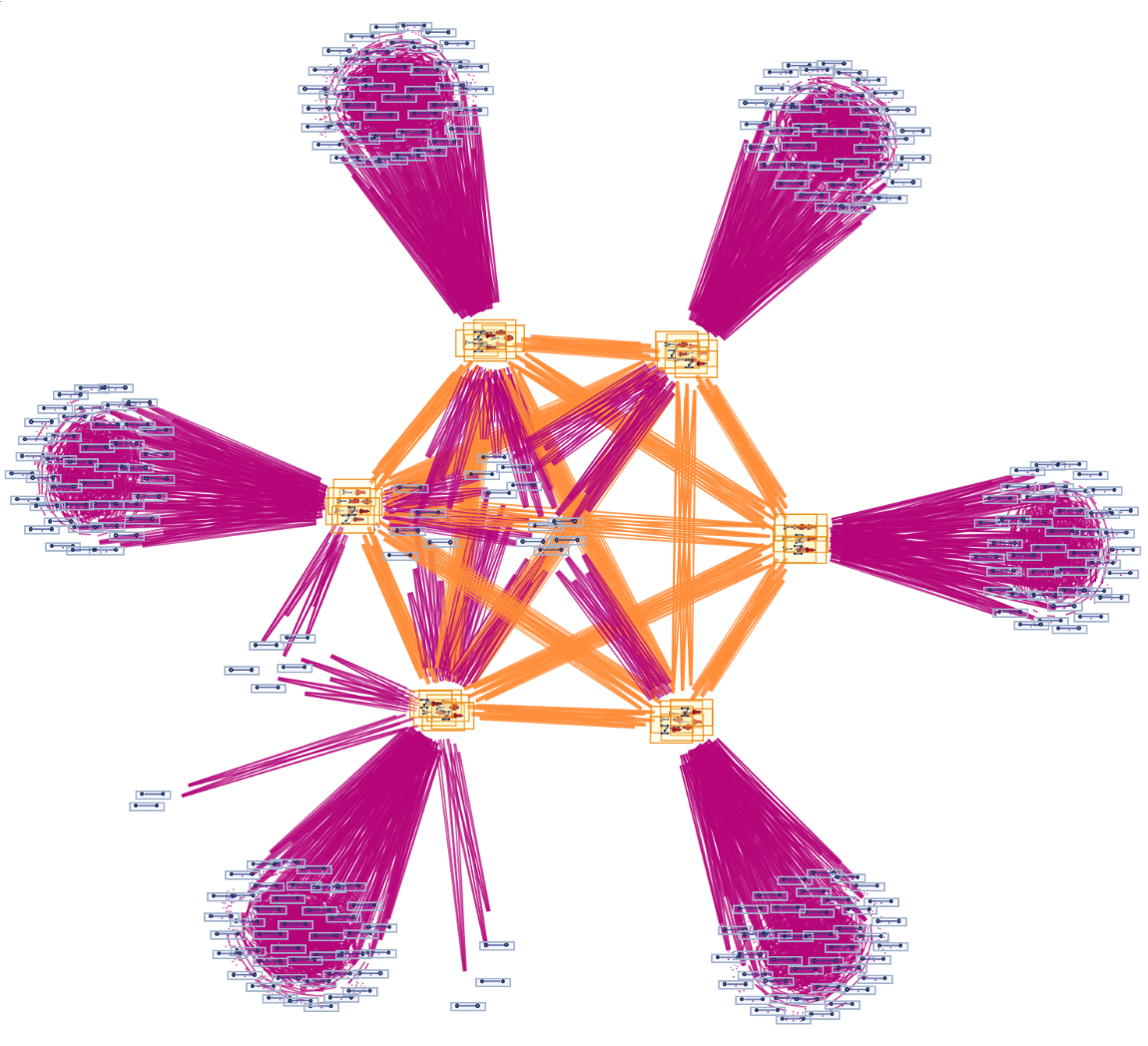Show just the structure of the graph, without labels:

 In:=Out=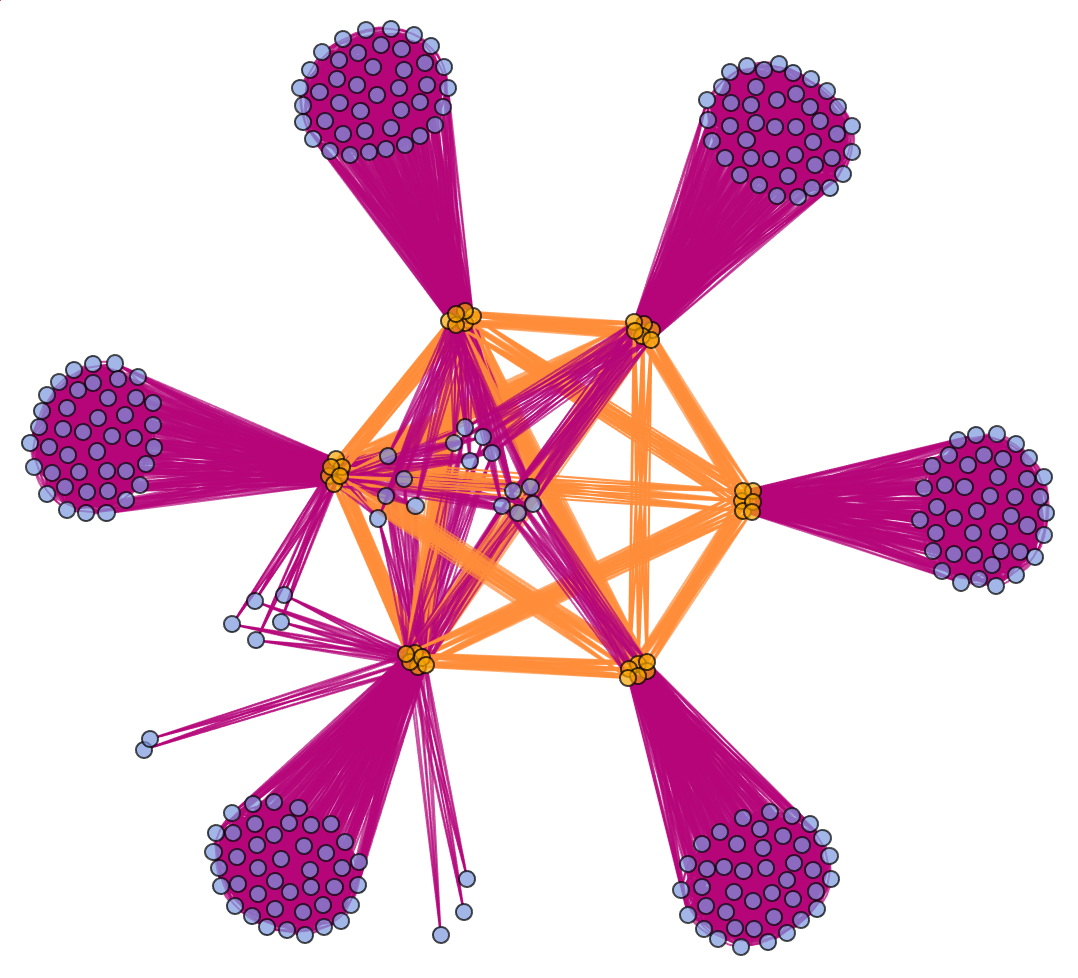Merge all instances of equivalent tokens that appear at each time step:

 In:=Out=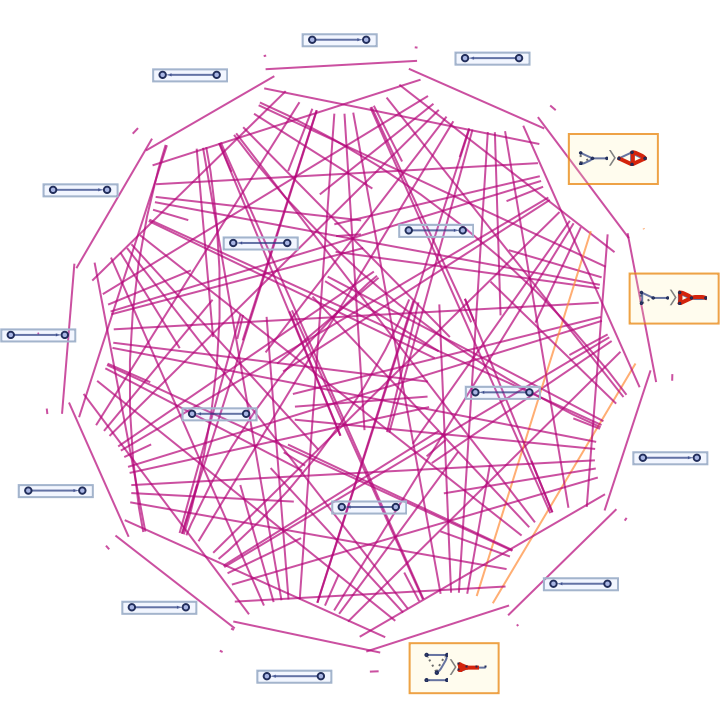Run the system for more steps:

 In:=Out=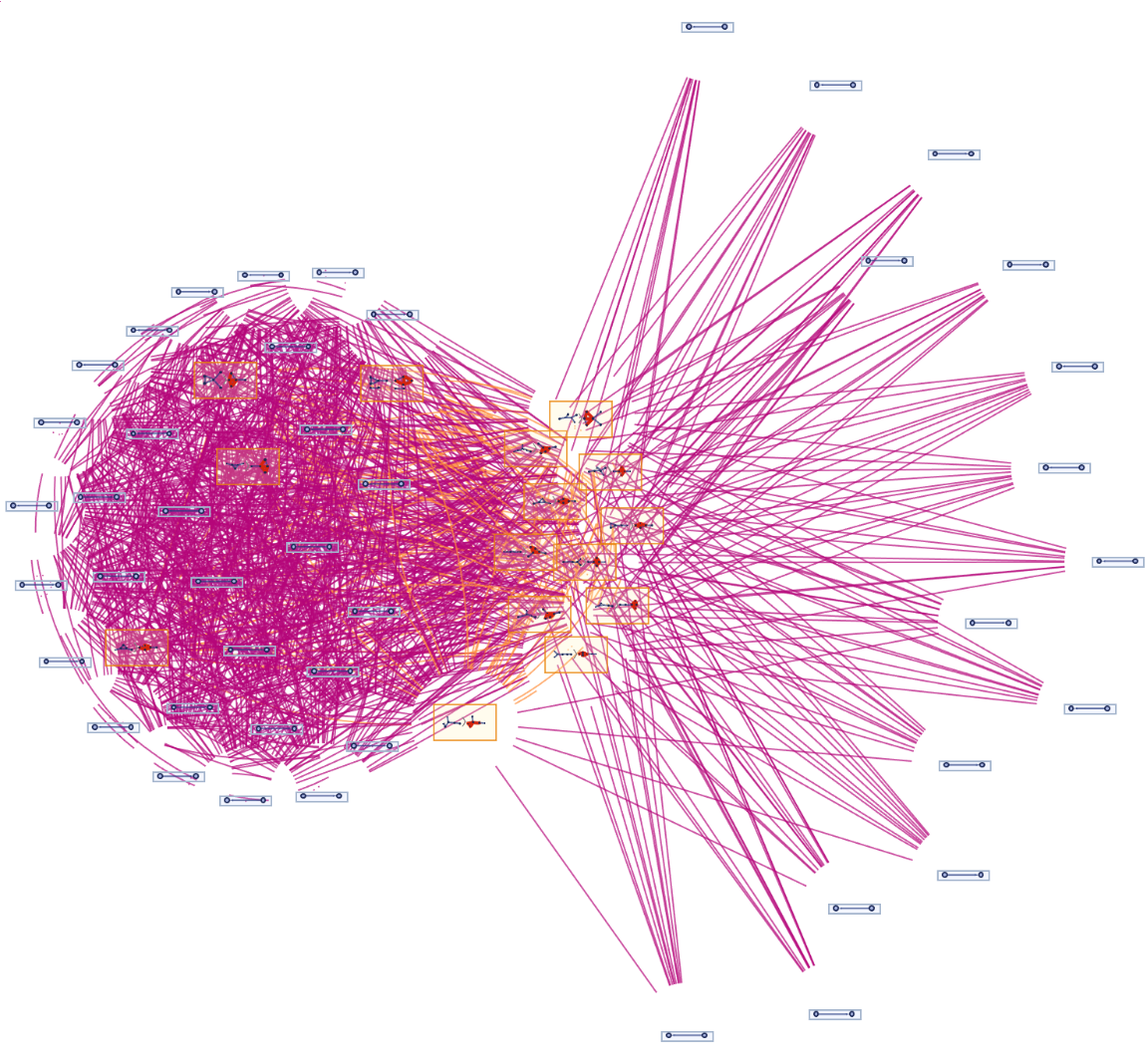Show just the structure of the graphs, without labels:

 In:=Out=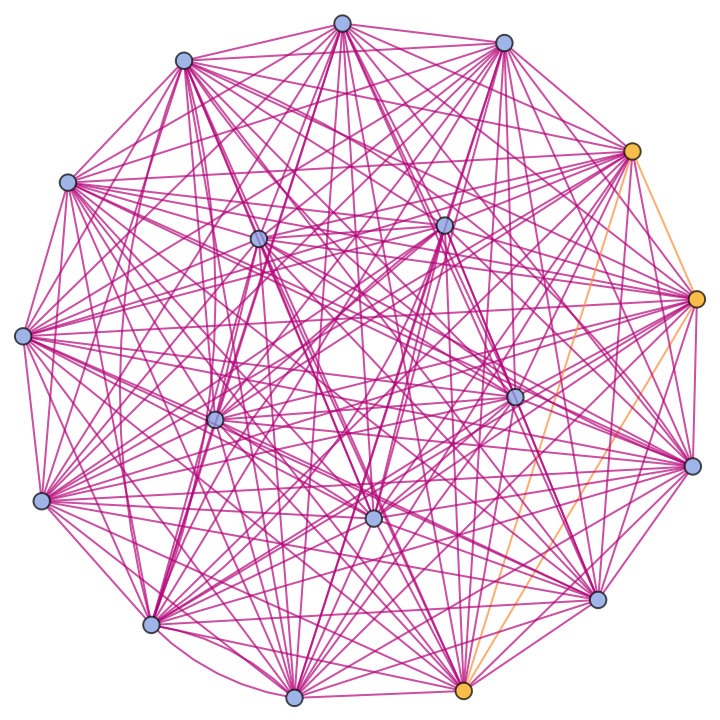In:=Out=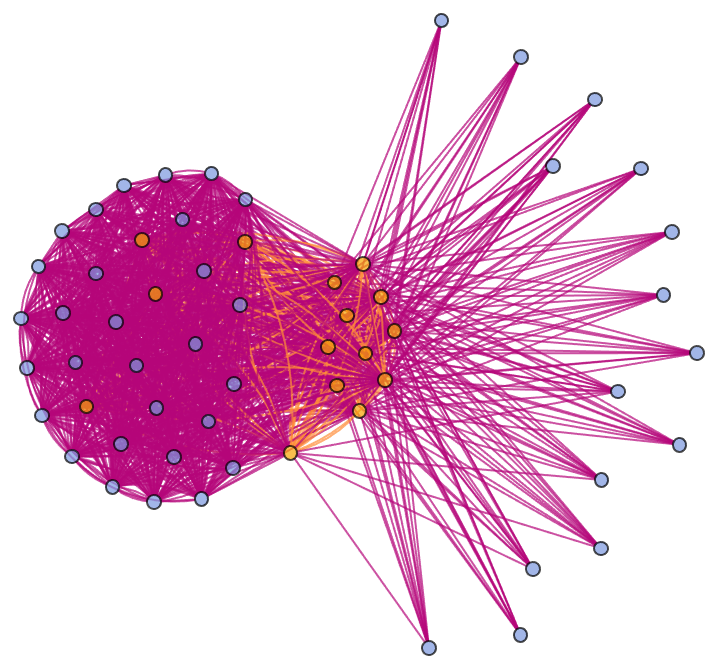Specify an event selection function that picks only up to two events at each step:

 In:=Out=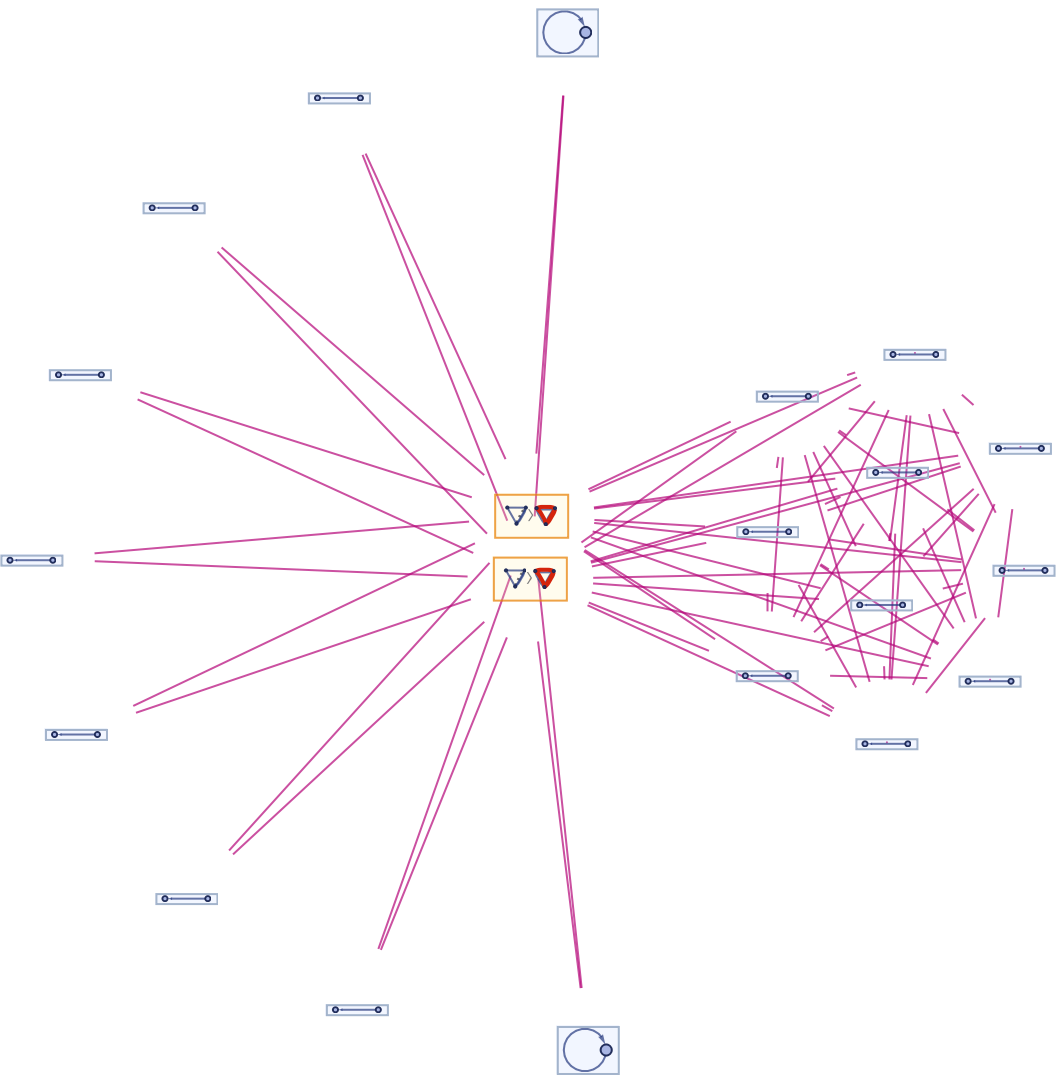### Scope (5)

#### Rules and Initial Conditions (2)

WolframModelGlocalBranchialGraph accepts both individual rules and lists of rules:

 In:=Out=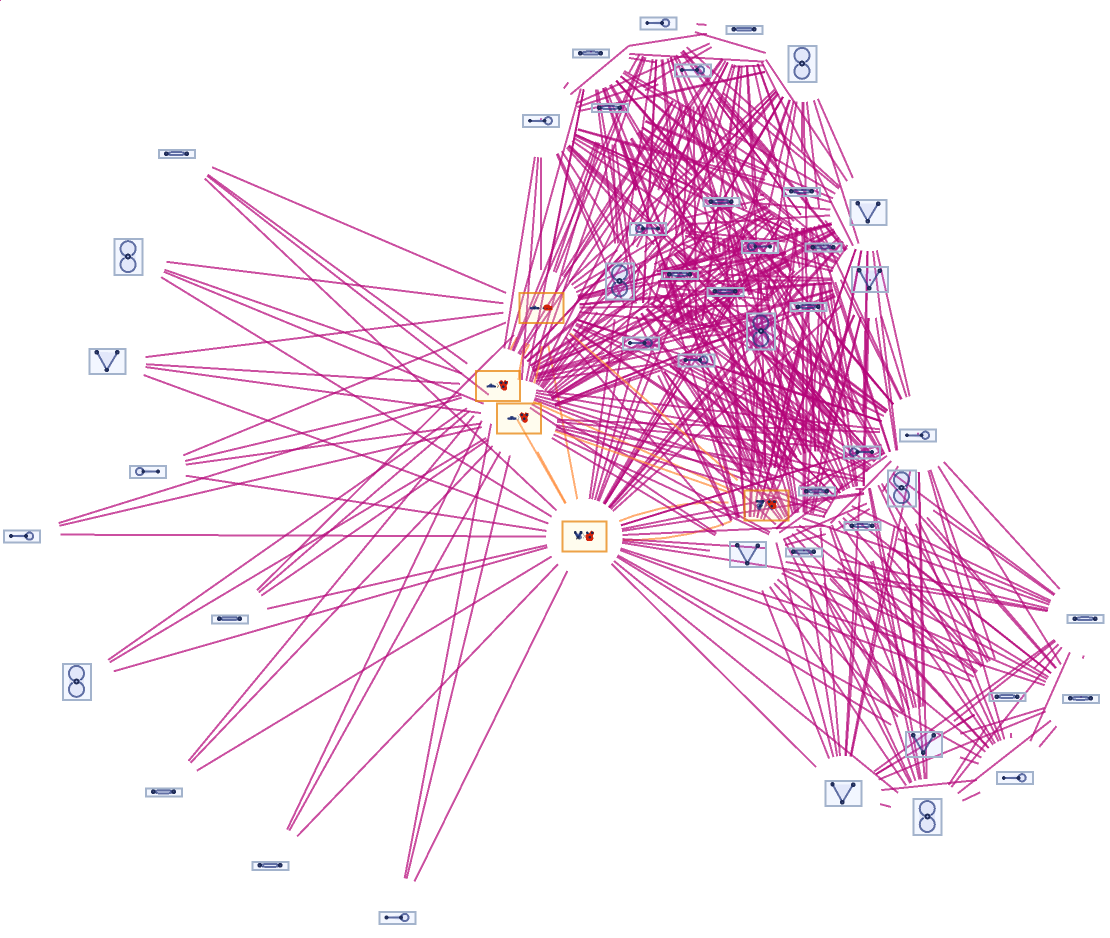In:=Out=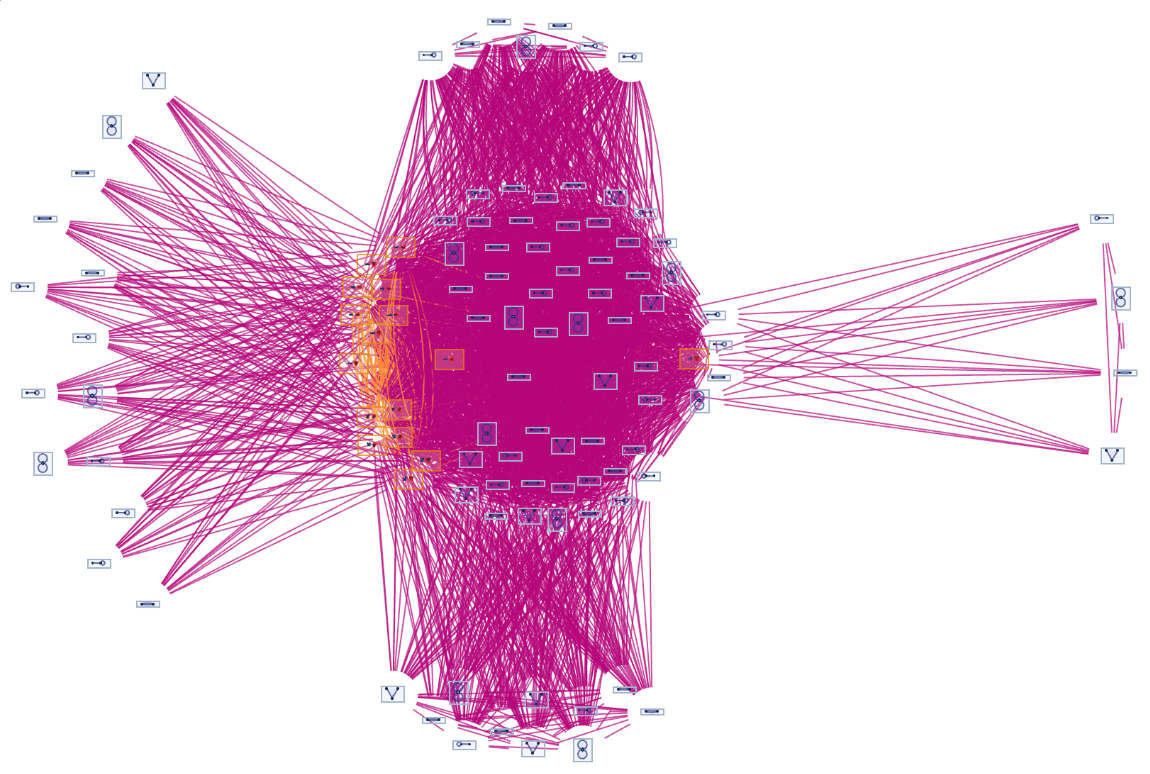Likewise for initial conditions:

 In:=Out=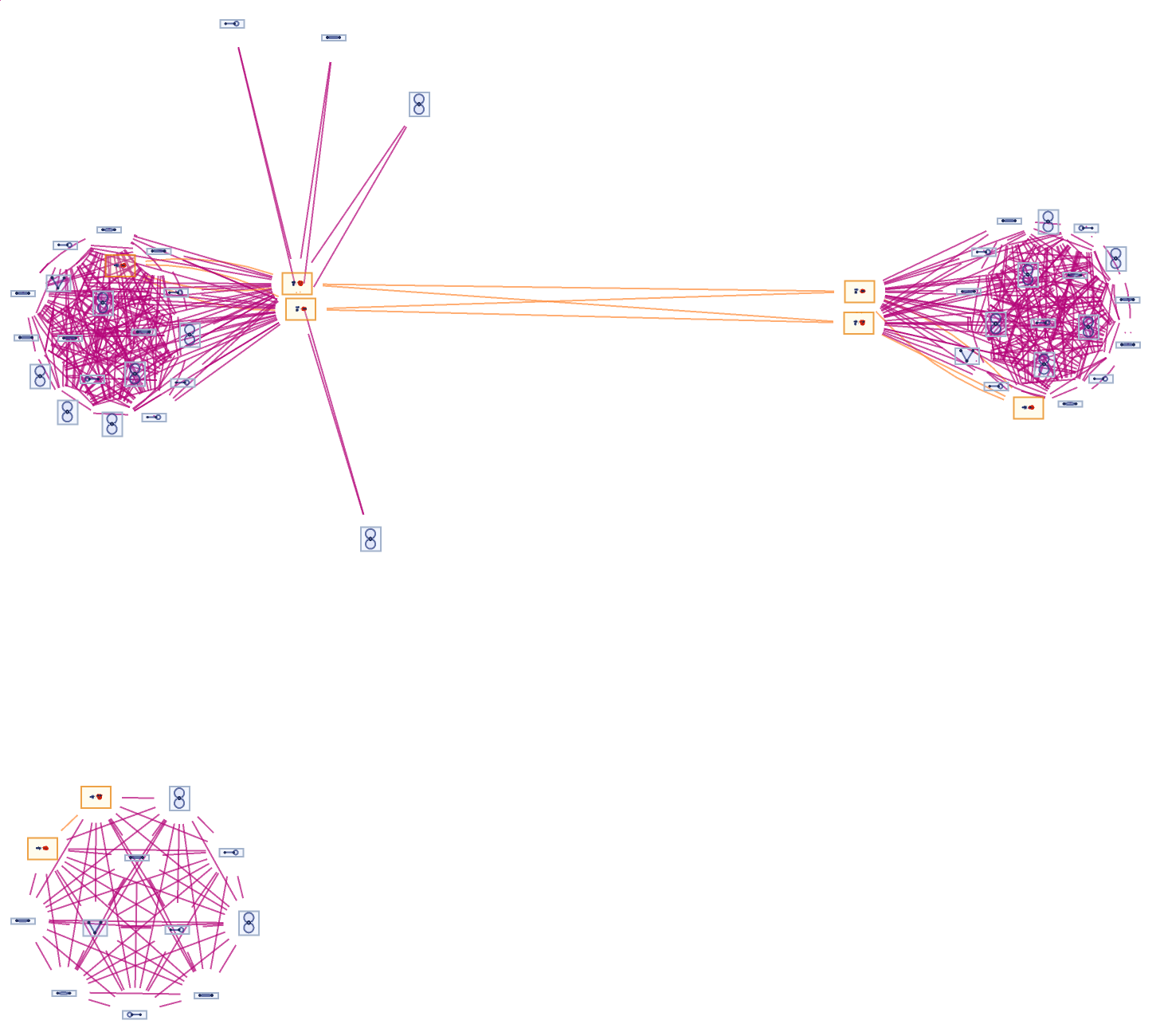#### Event Selection Function (3)

Apply only the first possible event at each step:

 In:=Out=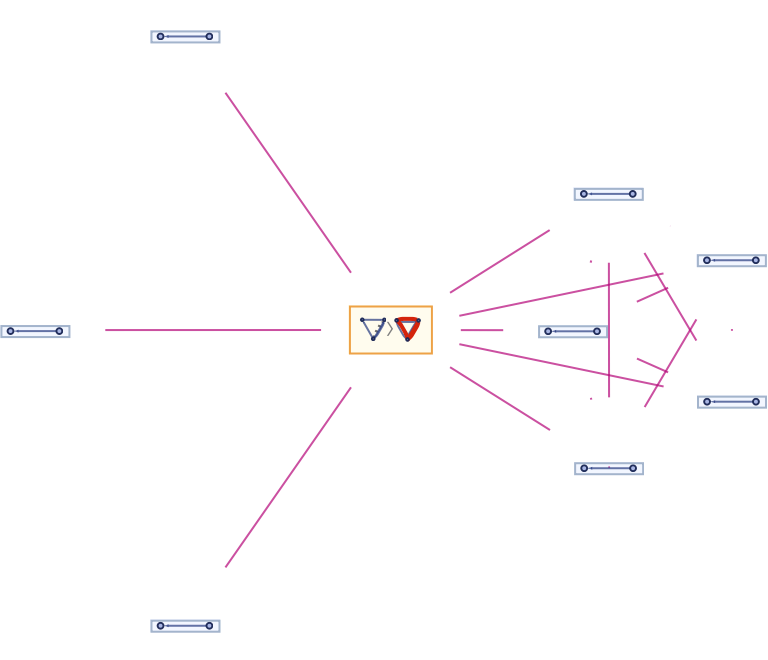Apply the first and last possible events at each step:

 In:=Out=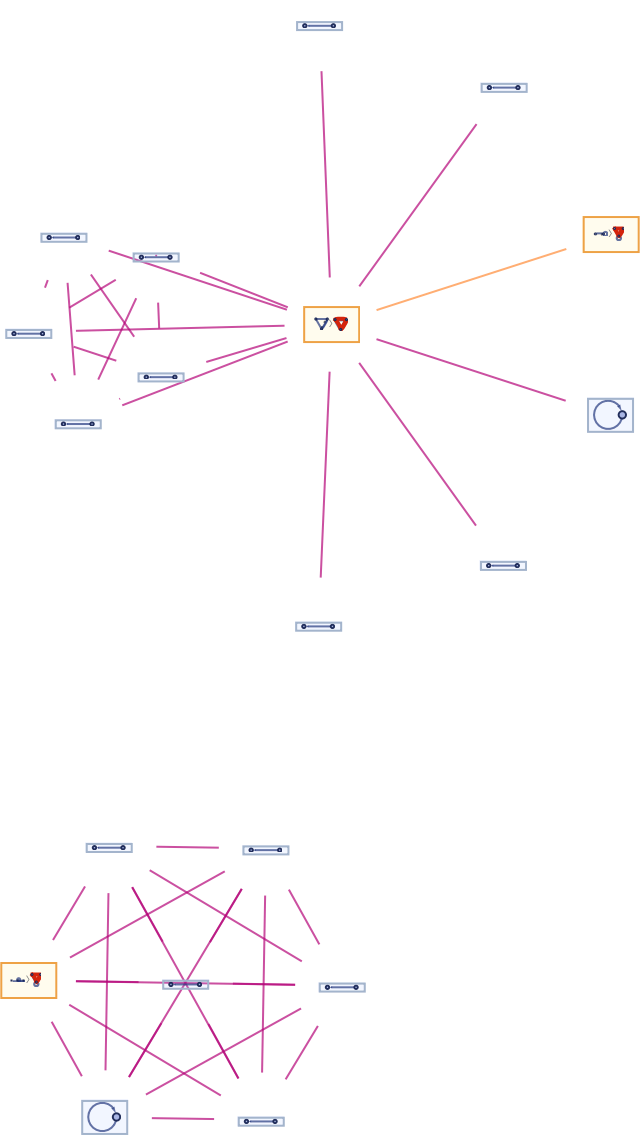Compare this to the full branchial graph for the unrestricted glocal multiway evolution:

 In:=Out=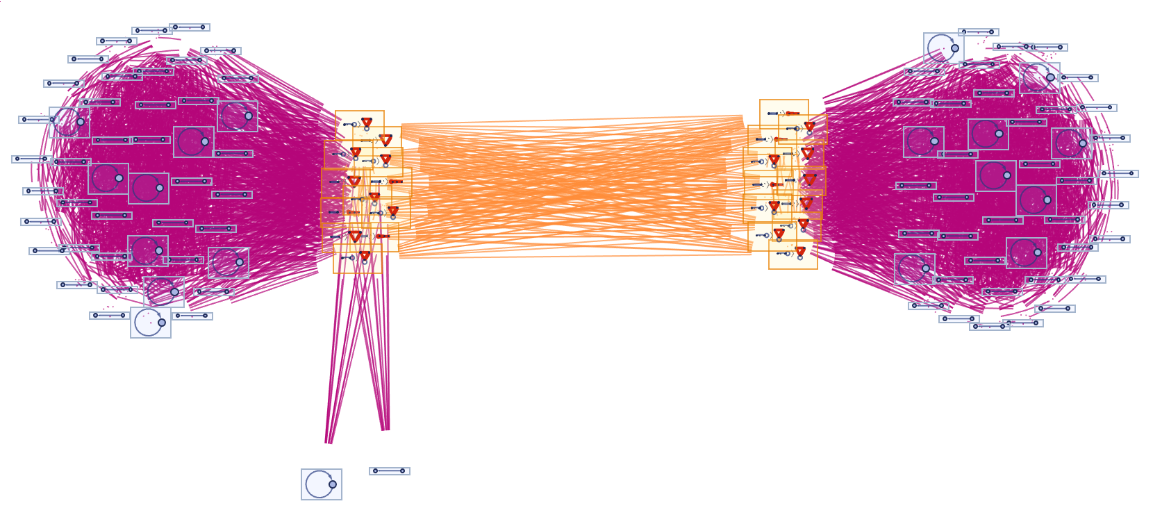### Options (15)

#### DeduplicateTokens (2)

By default, equivalent tokens remain unmerged at each time step:

 In:=Out=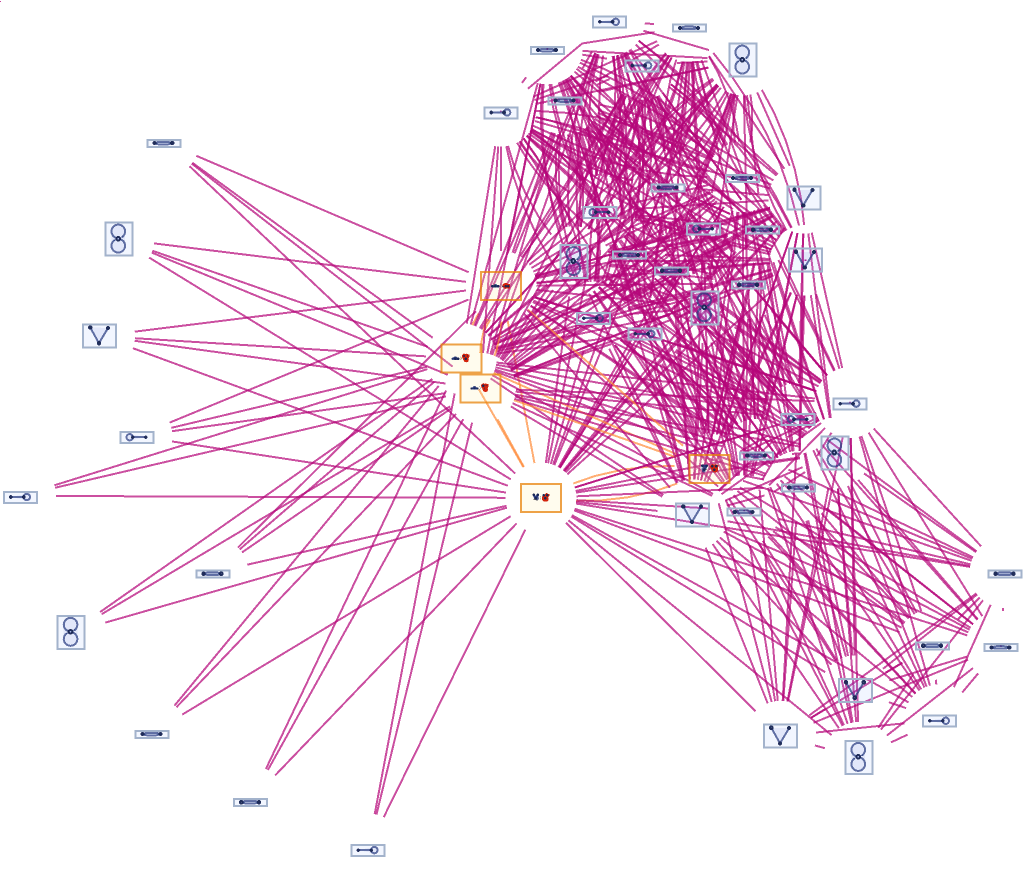Merging of equivalent tokens at each time step can be enforced using the option "DeduplicateTokens":

 In:=Out=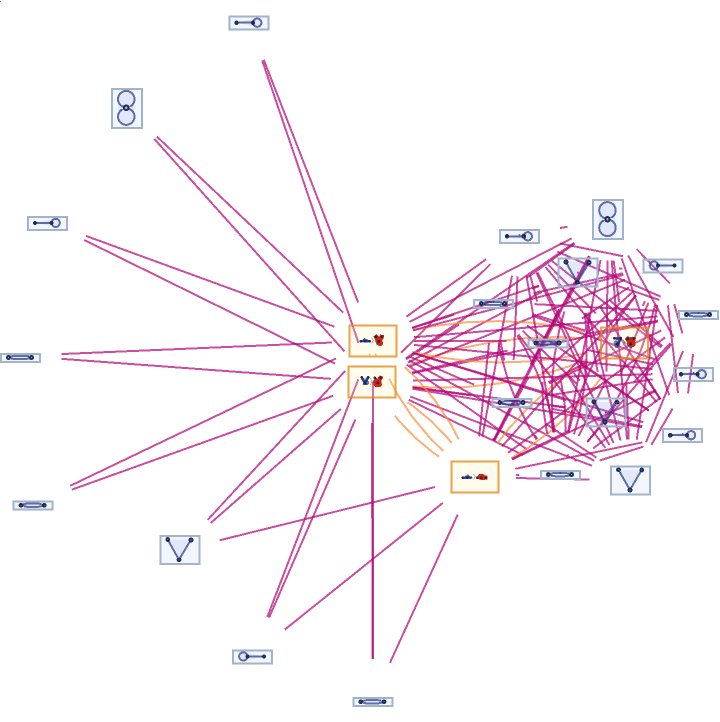#### VertexRendering (2)

By default, state/token vertices and event vertices use special rendering (inherited from the MultiwaySystem resource function):

 In:=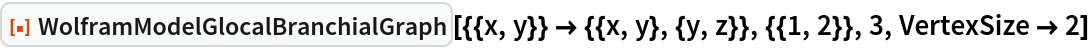Out=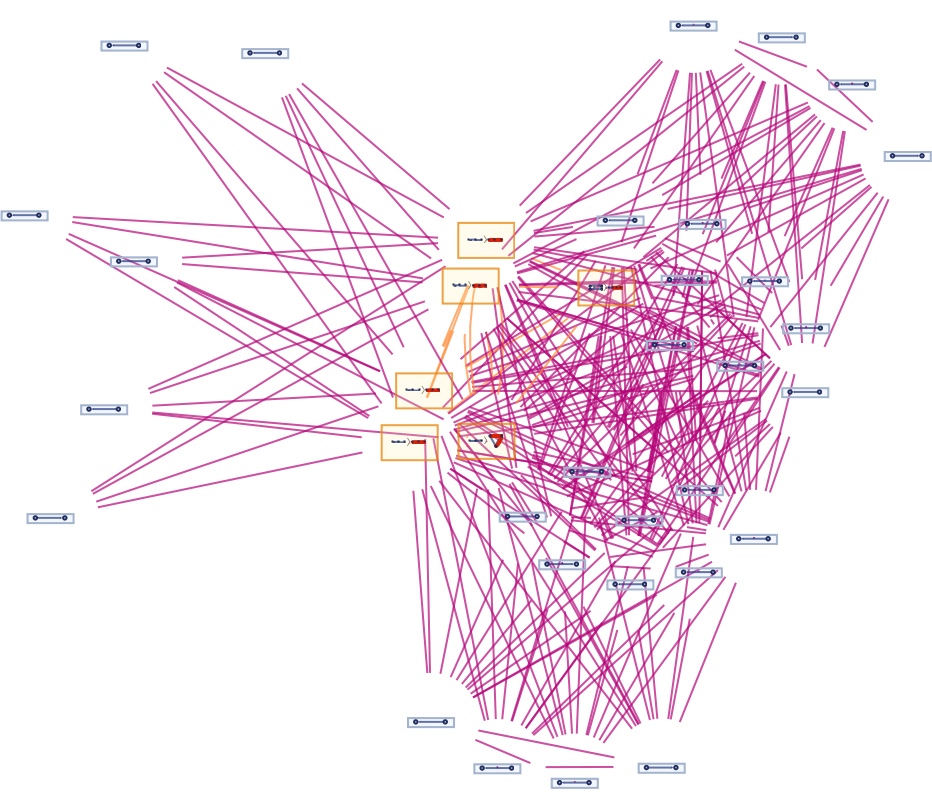This rendering can be disabled using the option "VertexRendering":

 In:=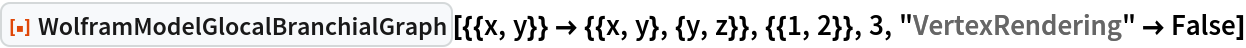Out=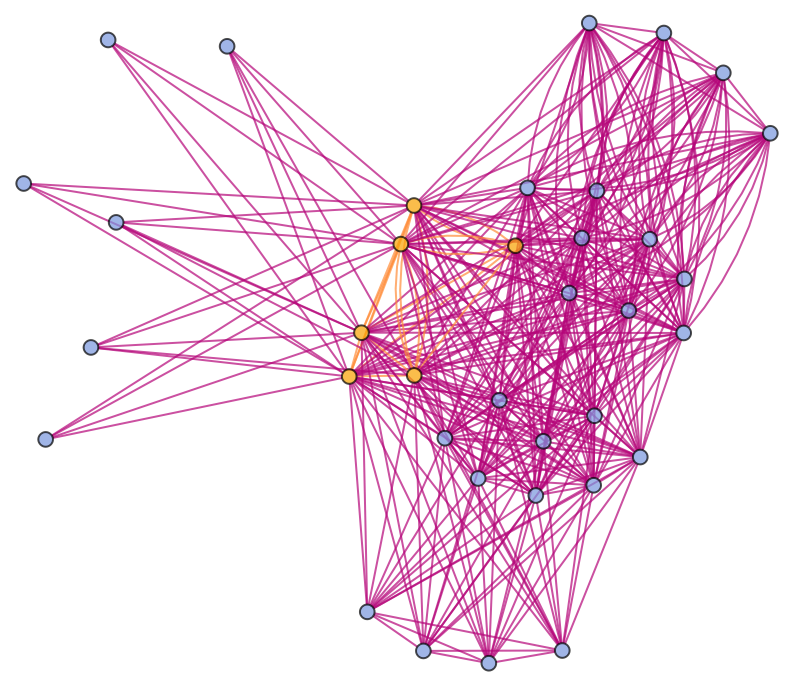#### StateRenderingFunction (4)

By default, states/tokens are labeled by their contents:

 In:=Out=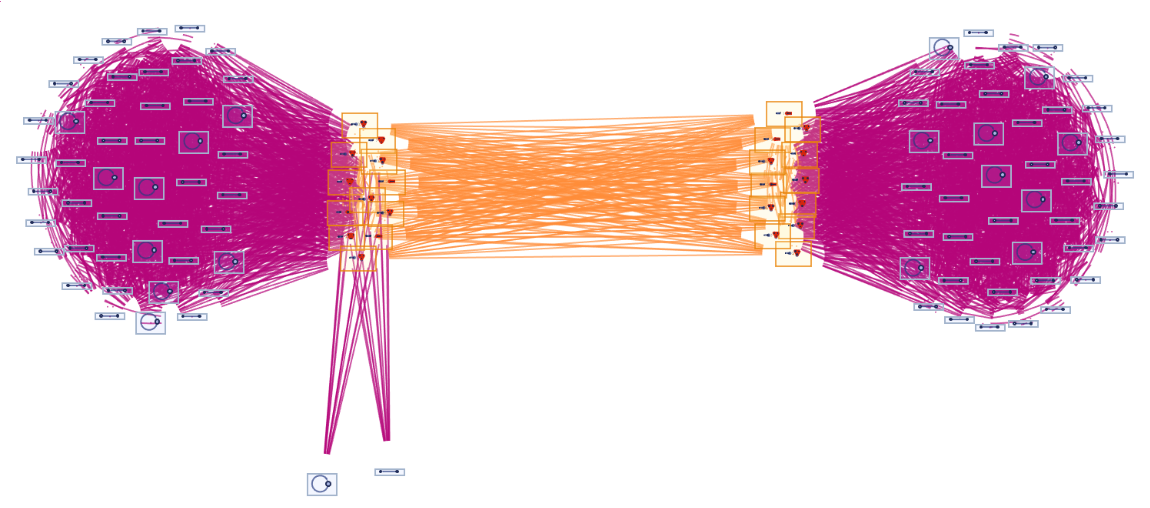Use no labeling for states/tokens:

 In:=Out=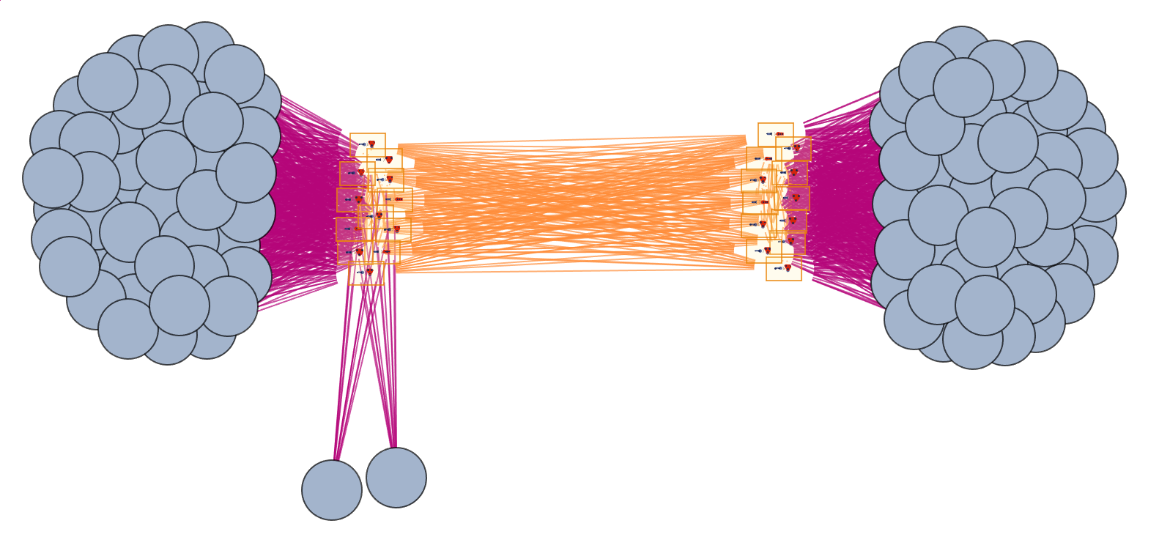Use raw state/token names as vertex labels:

 In:=Out=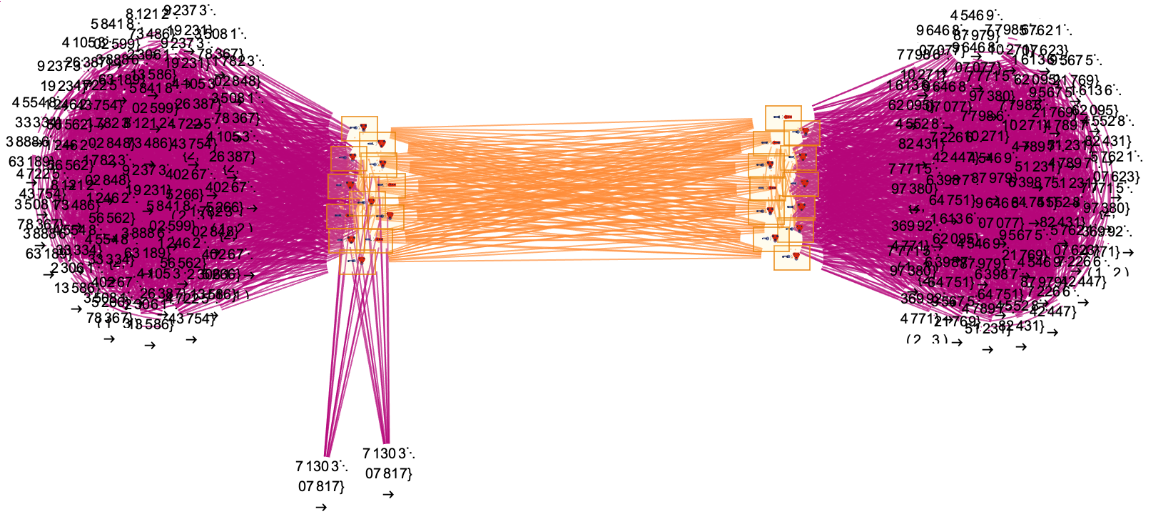Use a named shape as each state/token label:

 In:=Out=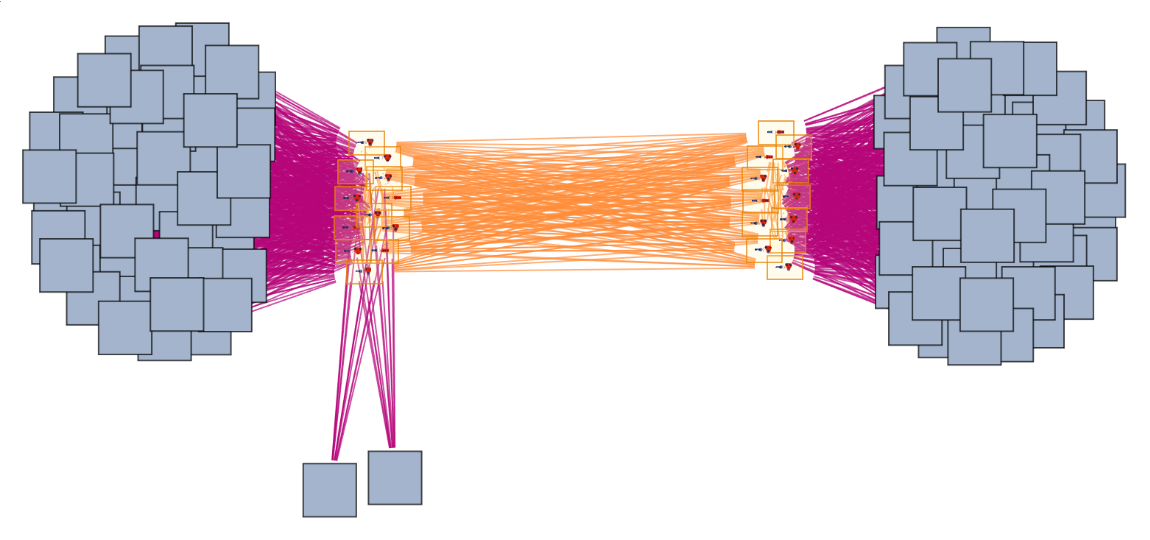#### EventRenderingFunction (5)

By default, both states/tokens and events are labeled by their contents:

 In:=Out=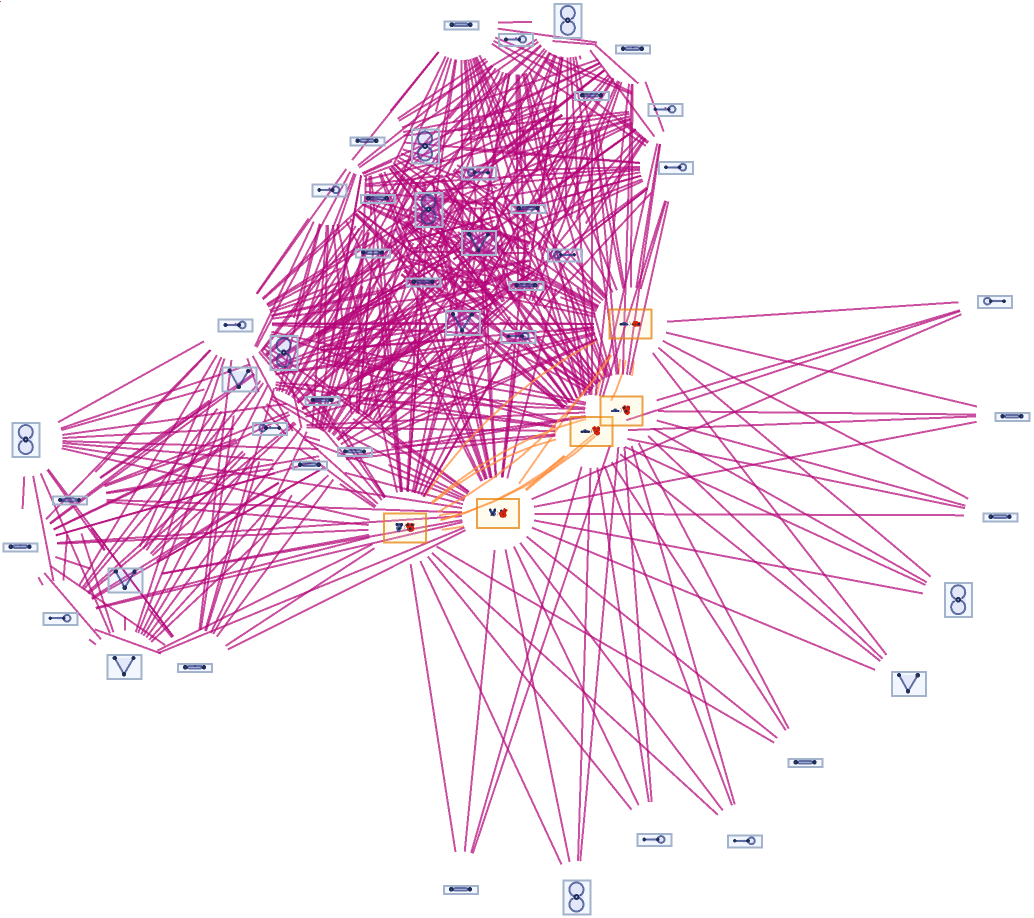Use no labeling for states/tokens:

 In:=Out=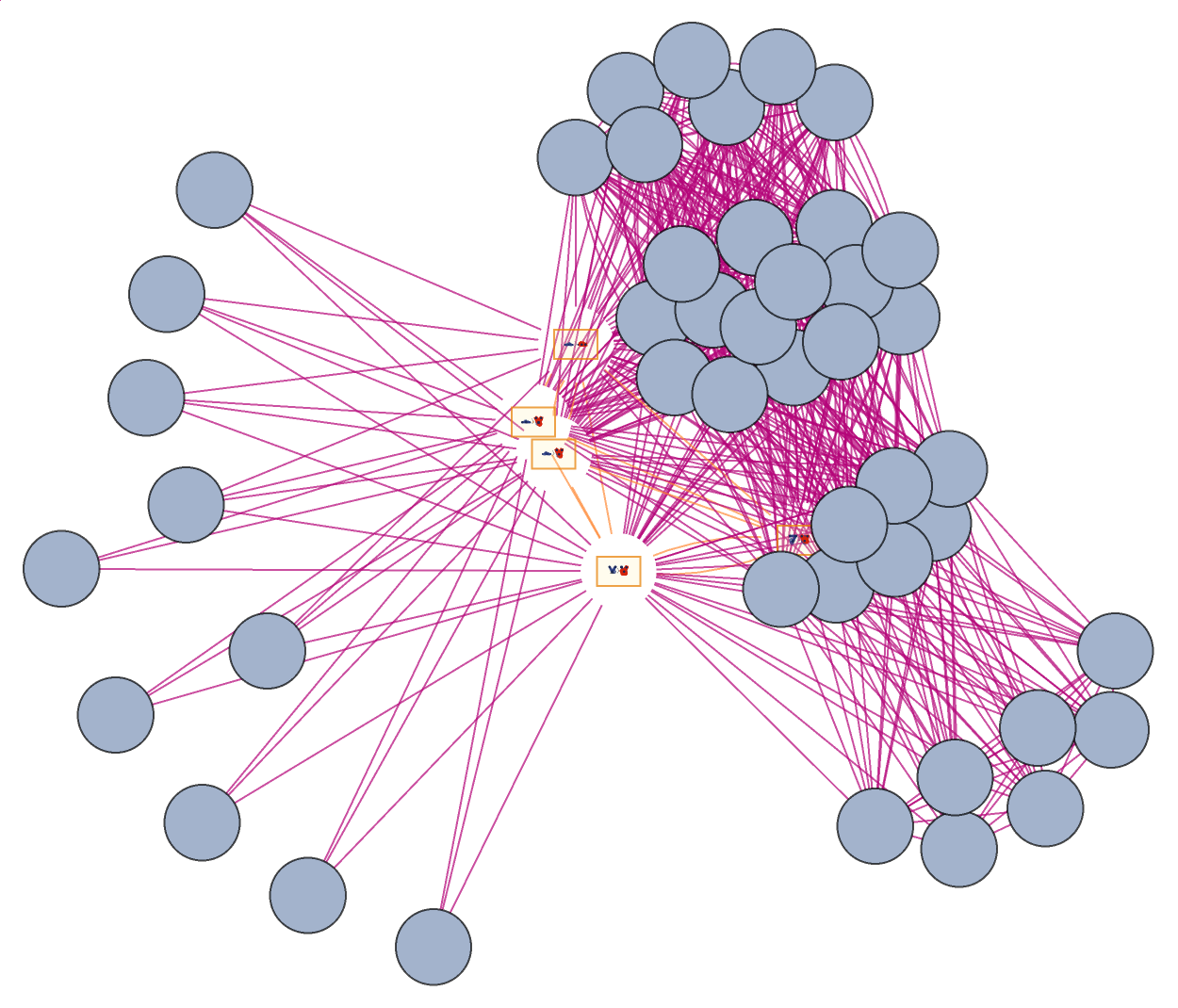Also use no labeling for events:

 In:=Out=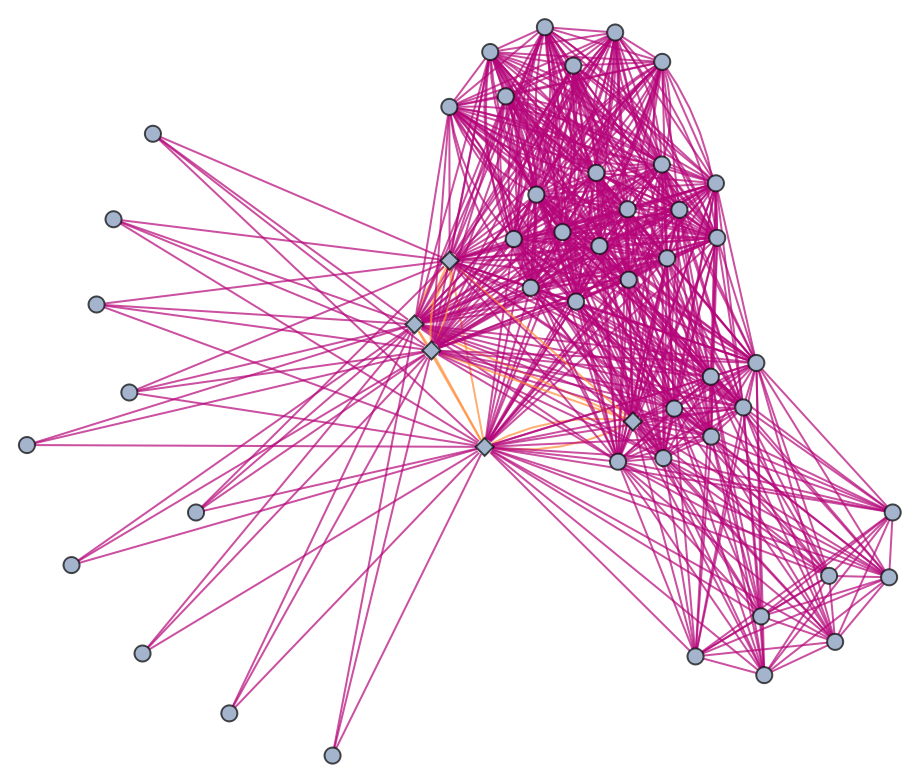Disabling vertex rendering yields an equivalent result:

 In:=Out=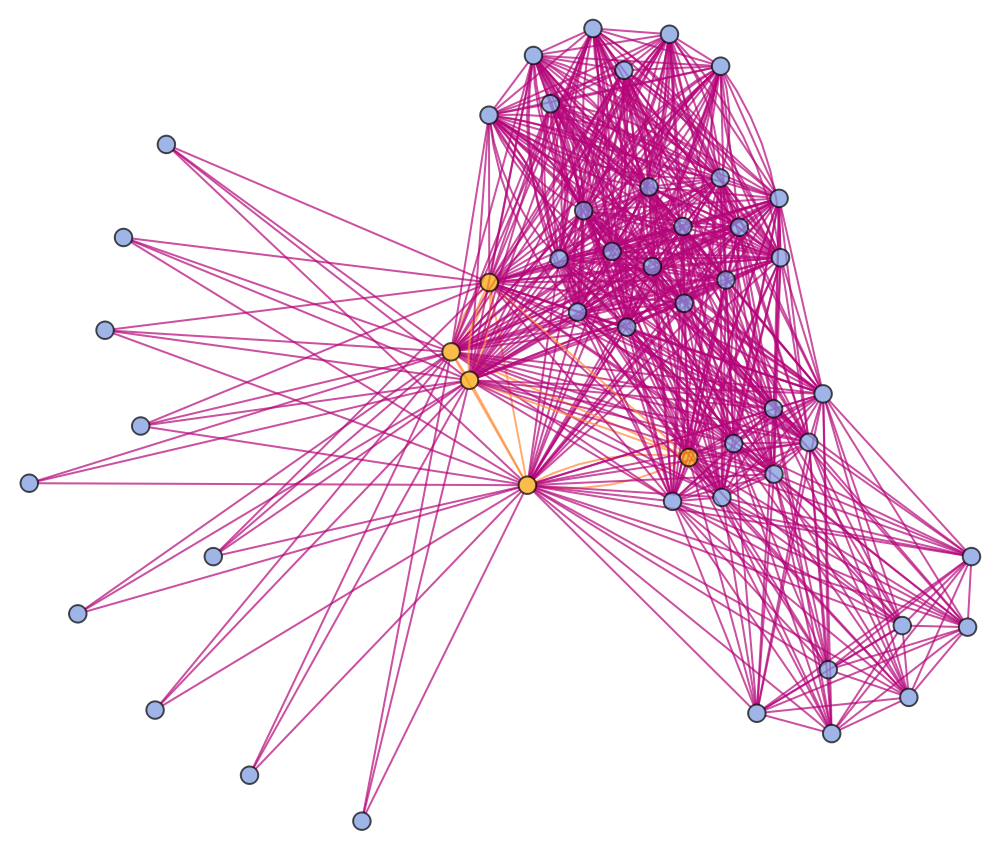Use raw event expressions as their labels:

 In:=Out=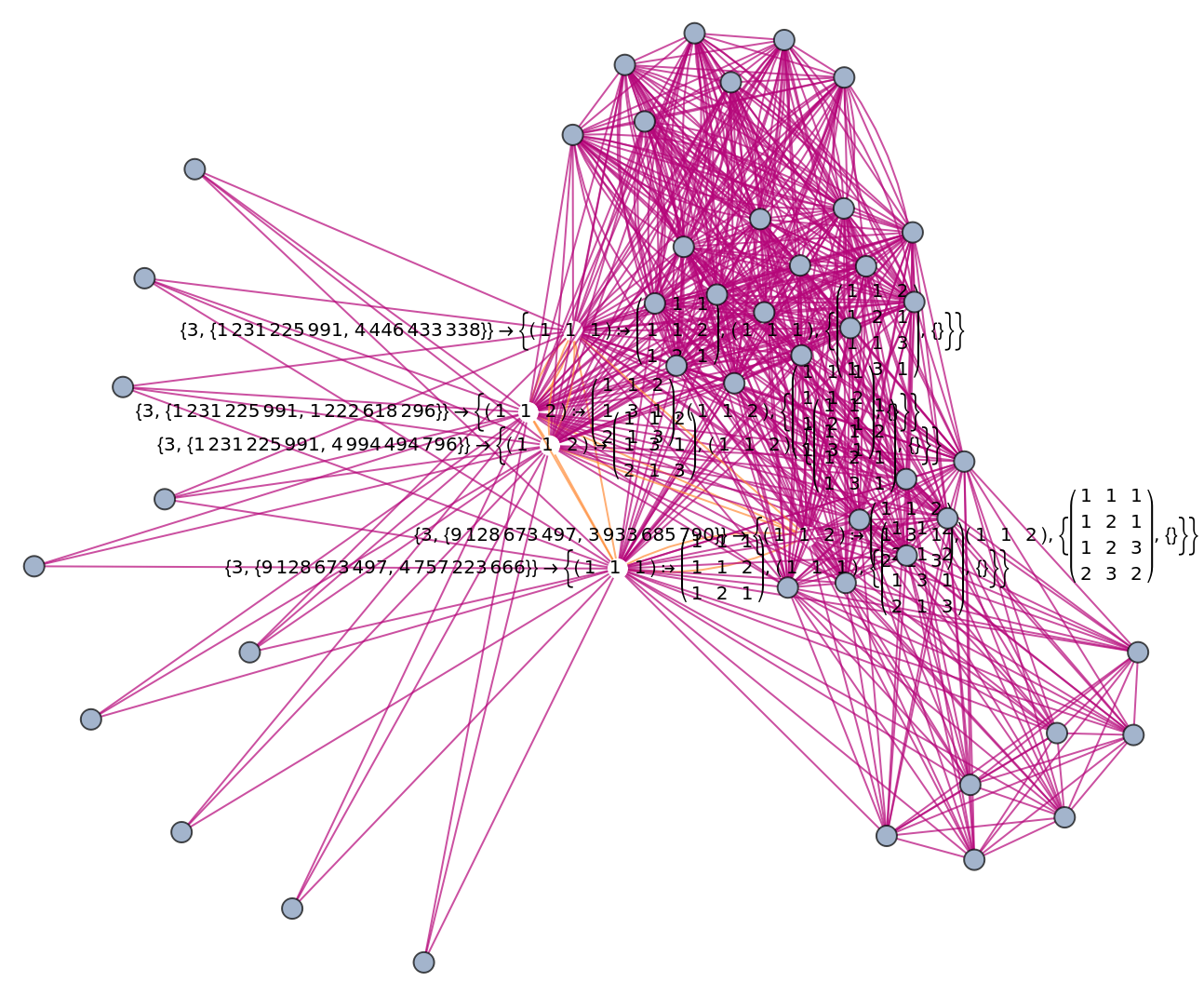#### GraphLayout (2)

Generate an example glocal multiway evolution:

 In:=Out=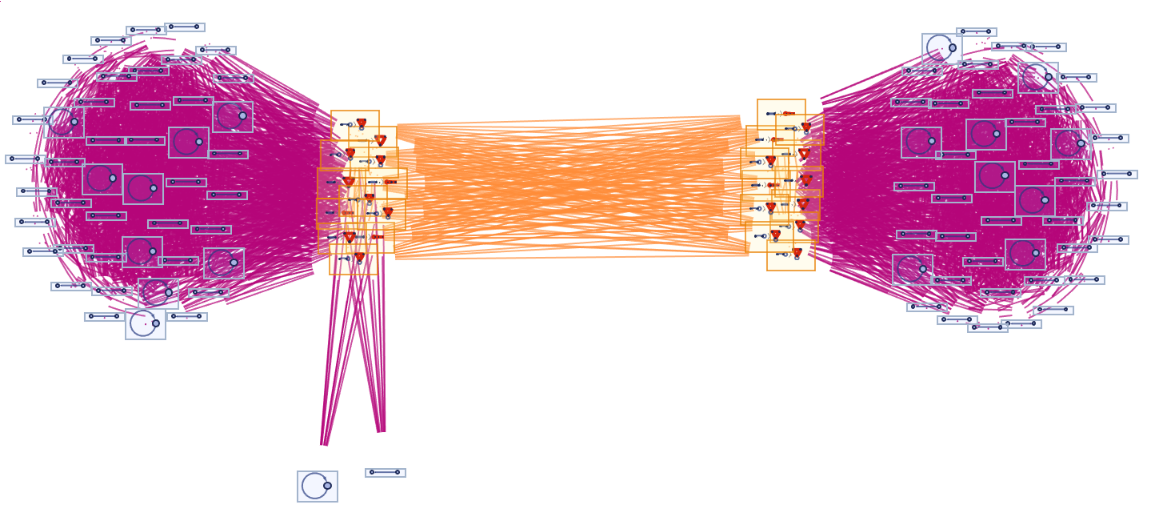Force a spring embedding (as opposed to the default spring electrical embedding):

 In:=Out=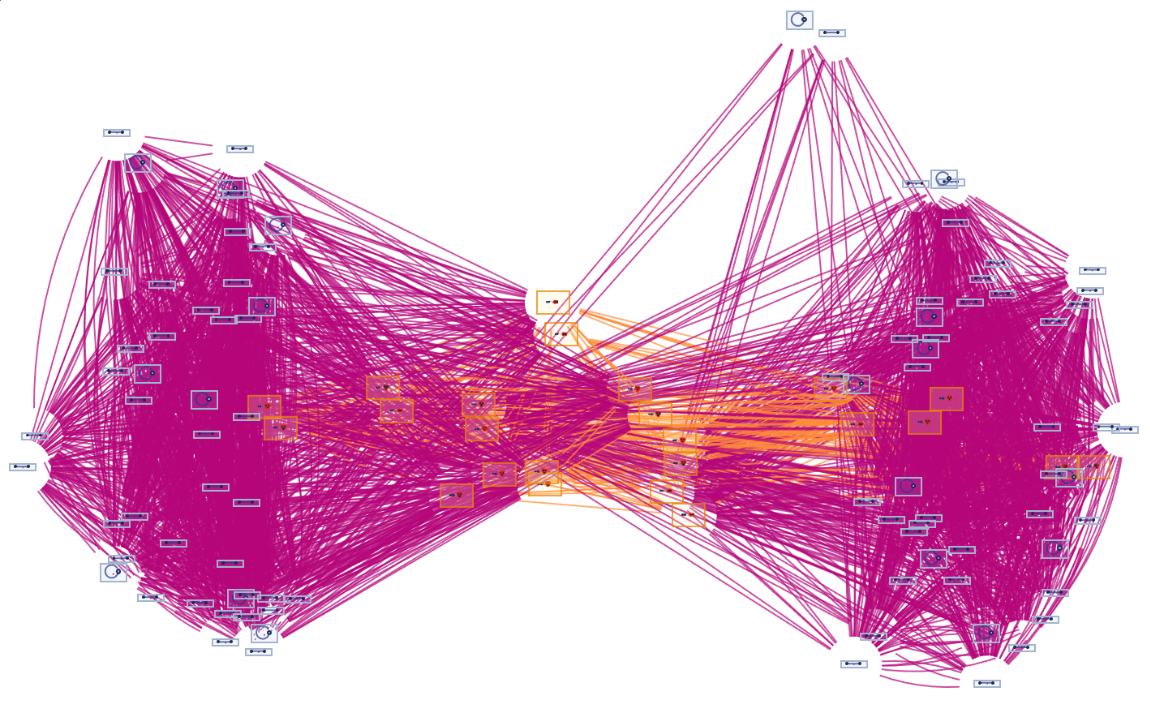Jonathan Gorard

## Version History

• 1.0.0 – 22 November 2021